### Introduction

Some time ago I was trying to write out the guitar part for a piece of music for bandoneon and guitar, and was having a great deal of difficulty - I could pick out the bandoneon tune, and the guitar bass, but hardly anything of the notes in between. Then I realised that I could use a wavelet analysis program (which I'd just written for use with chemistry data from an aircraft experiment) to work out exactly what notes were being played at each "instant" in time. From doing this, it's only a small step towards looking at the spectrum of the sound of the concertina...

### Spectra...

I've used as an example a snippet of solo concertina from Dave Townsend's recording, "Portrait of a Concertina". Here's an audio file file from the first tune on the first track, Lovesick Polly. Now let's have a look at the very first note, which is an "e", one-and-a-bit octaves above middle C. Here's a plot of the signal (which represents air pressure/movement of a loudspeaker etc) against time on the horizontal axis: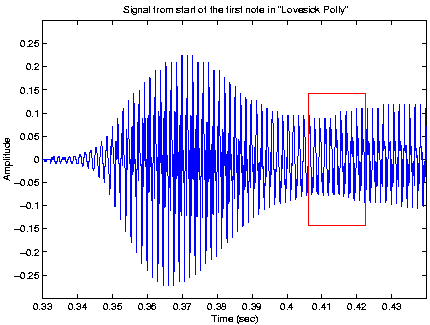Not all of the note is plotted - in total the note actually lasts around half a second. What you can see is that when Dave plays this (and most other) note(s) he starts the note with quite a strong push/pull on the bellows, then relaxes the pressure for the remainder of the note. In this case, the peak power at the beginning of the note is a little over four times the power during the rest of the note (as the power goes as the square of the amplitude). Take heed, all ye concertina players who keep a constant pressure on the bellows because if you want to sound like Dave Townsend. then you have to think about the bellows as well as the notes!

Now if we zoom into the region outlined by the red box in the plot above, we can see the waveform itself: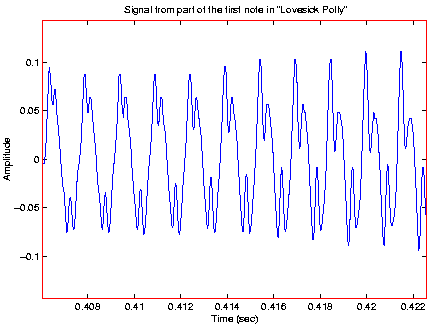You can see that this consists of one dominant smooth wave, which has a couple of "wiggles" superimposed on it. The main wave is the fundamental note, with a frequency of 660 Hz. The wiggles are caused by the harmonics, and are what give any instrument it's distinctive "tone".

If we want to see exactly what notes these harmonics correspond to, then we can do a Fourier analysis of the signal. The result of doing this on just the "middle" of Dave's "e" note (i.e. ignoring the middle and end of the note, which would just complicate things) we get a plot like this: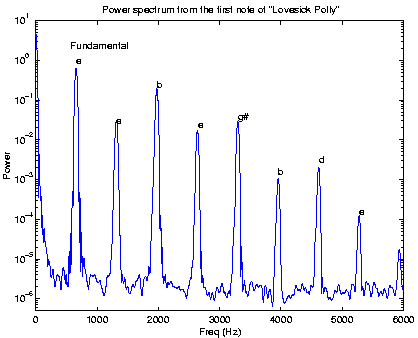On the vertical axis is power (i.e. how loud the note/harmonic sounds). On the horizontal axis is frequency. Each vertical peak (apart from the one at 0 Hz) corresponds to either the fundamental note (at 660 Hz), or a harmonic, and the height of each peak indicates how loud it is. The pitch increases to the right of the plot, and the note name is indicated next to each peak.

What this shows is that the harmonic (1320 Hz) one octave above the fundamental note being played is around 5-6 times quieter than the fundamental itself (if the relative loudness goes as the ratio of the logarithm of the powers). What I found quite surprising is that the next harmonic, which is a "b" one-octive-plus-a-fifth above the fundamental, is only a factor of 3 times quieter than the fundamental. Has anybody got any ideas why this might be the case? I'd be quite interested to compare the spectrum above with other instruments... Also, if one reconstructed a signal with just the peaks (i.e. forgetting about the "noise" in between), would it sound like a concertina?

### Wavelets...

Rather than simply obtaining one spectrum for one time "window", we can obtain lots of spectra by analysing the signal with many very narrow windows - this is like looking at the plot at the top of this page with tunnel vision, and working out what frequencies are sounding at any one instant (plus/minus a small fraction of a second). This is basically how wavelet analysis works. If we do it to the first three seconds or so of "Lovesick Polly" we get a plot that looks like this: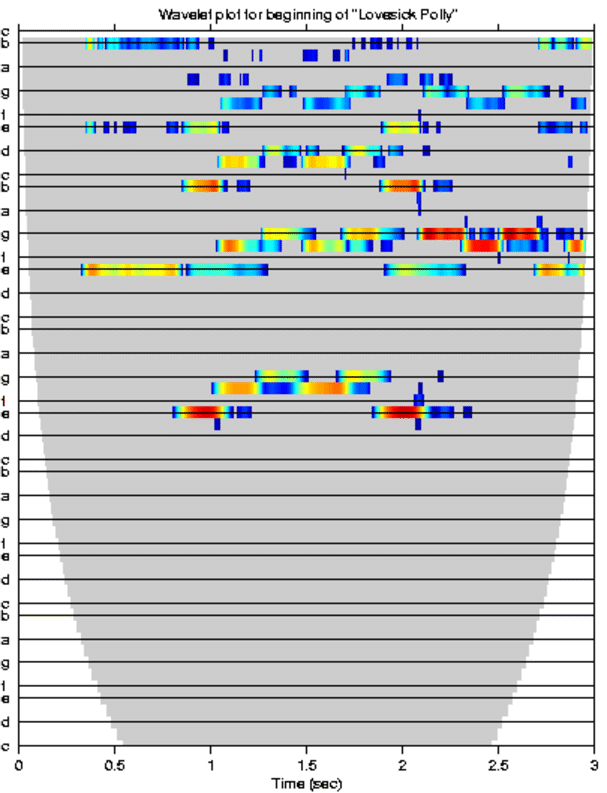Time goes across the plot from left to right. Frequency increases as we go up the plot, though the axis has been marked with the note name. The intensity of the sound is indicated by the colour, with blue indicating "quiet" and red indicating "loud". If you only concentrate on the notes nearest the bottom of the plot (i.e. the fundamentals) at any given time, you can see how it gives you the tune: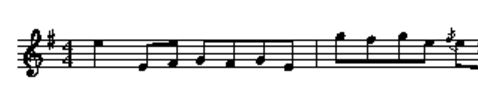so if you're trying to work out how a really fast/difficult tune goes, a wavelet analysis might help!

The problem is that the harmonics are really quite strong, especially the octave-plus-a-fifth one, so when you've got more than one instrument (especially if it's a 3-voice bandoneon + guitar!) it's often quite difficult to distinguish between the fundamental notes and all the harmonics. Perhaps, if you know only one instrument is playing (e.g. concertina), it would be possible to scan "up" the wavelet diagram above, and remove the higher harmonics in proportion to the first significant note that you come across,,,,? Even so, the wavelet analysis as it is can still let you quite easily transcribe multi-part music (you have to "subtract" the harmonics in your head...), such as Dave Townsend's arrangement of Stains Morris: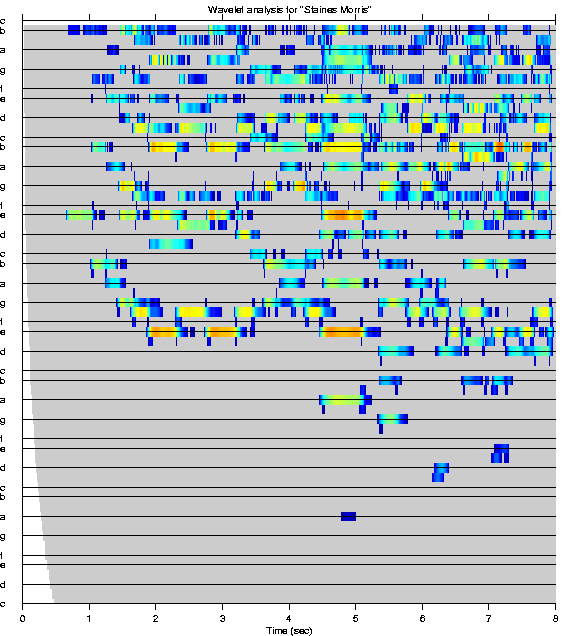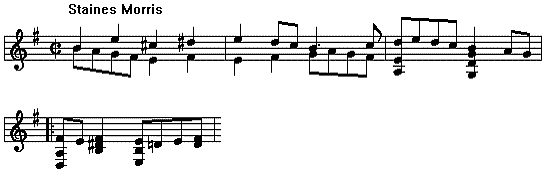There are a couple of things I'd like to try, such as subtracting harmonics as described above, which might make the technique easier to use for transcribing music. I'd also like to see whether it's possible to change the speed/pitch of music by stretching it in "wavelet space", before then transforming it back. If anyone has any ideas, let me know!

Here's a copy of the wavelet code, for you entertainment:

• Some MATLAB code for reading in an audio file, preparing variables, running the wavelet function (below), and plotting the result
• The wavelet routine (Fortran). It's set up as a Matlab MEX file, but would be easy to convert to a stand-alone routine etc...
• The same routine in C (note, it runs slower than the Fortran code, even though I tweaked things to try and make it run faster...)
• The wavelet routine isn't very well written, as it uses up far more memory than necessary. This would be easy to fix.

Back home